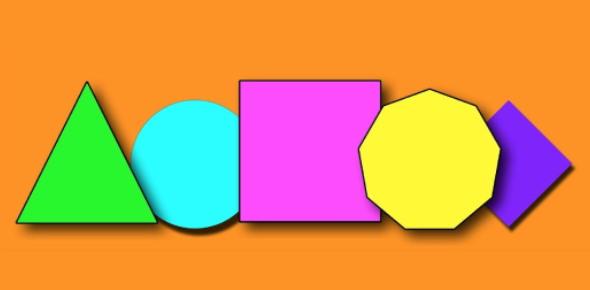# Geometry General Questions! Trivia Test! Quiz

9 Questions | Attempts: 4034
ShareSettingsThe trivia test quiz below is made up of some general geometry questions! Different shapes have different angles, and when you know how the angles are elated, you might actually know different shapes. How about you test out your geometry understanding by taking this all exciting but challenging quiz. Check it out and keep an eye out for others like it to perfect your geometry skills. All the best!

• 1.
Two triangles with equal sides are considered _____________.
• A.

Similar

• B.

Congruent

• C.

Isosceles

• 2.
A triangle with two equal sides and one non-equal side is called ____________.
• A.

Isoscelese

• B.

Scalene

• C.

Equilateral

• D.

Right

• 3.
A polygon with five sides is called a ____________.
• A.

Hexagon

• B.

Octogon

• C.

Pentagon

• D.

Trapezoid

• 4.
Two angles whose measures have a sum of 180 degrees are called ___________.
• A.

Supplimentary

• B.

Acute

• C.

Right

• D.

Complimentary

• 5.
A circle has a total of how many degrees?
• A.

90

• B.

180

• C.

360

• D.

540

• 6.
A triangle has a total of how many degrees?
• A.

90

• B.

180

• C.

360

• D.

540

• 7.
Two angles whose sum is 90 degrees are called _________.
• A.

Supplimentary

• B.

Right

• C.

Acute

• D.

Complimentary

• 8.
A regular polygon has ___________.
• A.

All equal sides

• B.

All equal angles

• C.

A and B

• D.

None of the above

• 9.
Two lines with a common endpoint and vertex for a/an ____________.
• A.

Ray

• B.

Angle

• C.

Polygon

• D.

Line Segment

## Related TopicsBack to top
×

Wait!
Here's an interesting quiz for you.